# How Strong of a Linear Actuator Do I Need?

## What Am I Looking For?

The strength of a linear actuator is the amount of force it can deliver. It is typically seen in terms of Newtons (N) for metric units and pounds (lbs) for imperial units. There are two types of force specifications that linear actuator manufacturers will provide: Dynamic and Static.

Dynamic Force (or dynamic load) is the maximum force the actuator can apply to move an object.

Static force (or static load) is the maximum weight the actuator can hold when it’s not moving.

These force specifications are generally key factors in determining what linear actuator you need for your project. If you don’t know what other factors you might want to consider when selecting a linear actuator, check out our post about this here.

When trying to move an object with a linear actuator, you will need to determine what is the minimum dynamic force your linear actuator can have. This force will depend on more than just the amount of weight you are trying to move, but also the number of actuators involved and the physical geometry of your design. To determine the exact force requirement in any one application, you’ll need to apply Newton’s first law of motion. This law states that an object at rest tends to stay at rest unless acted upon by an unbalance force. For us, this means the force from our linear actuator needs to be greater than the sum of all forces acting against our desired direction of motion. This guide will walk you through how to calculate the forces involved using some basic examples.

Quick Aside: Free body diagrams are simplified diagrams of objects that are used to visualize the forces being applied to it. Utilizing these diagrams are good practice to visualize all the forces involved and their orientation.

## One-Dimensional MotionThe simplest case of utilizing a linear actuator to provide motion is using one actuator to move an object along one axis. As shown in the free body diagram next to this paragraph, the force applied by the linear actuator is label as F and the weight of the object is label as W. To determine the dynamic force required fro the linear actuator, you simply subtract the sum of the forces in the negative directions from the sum of the forces in the positive direction, which needs to be greater than zero to result in motio\n. For this example, it becomes F – W > 0. Then you need to solve for F, which becomes F > W.  This means that dynamic force requirement from the linear actuator needs to be larger than the weight of the object.

In a case where you are utilizing more than one linear actuator, like in the free body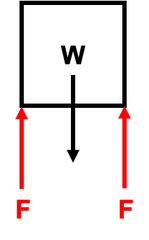diagram shown here, you follow the same process as above. For this example, the summation of forces becomes F + F – W > 0 or 2*F – W > 0. Then solving for F becomes F > ½*W. This means the force applied by one actuator can be less than the weight of the object, but the total force from both needs to be larger.

## Friction

The above cases ignored friction in their force balance calculations, which may or may not be the case in your application. The amount of friction force (f) is equal to the coefficient of friction (u) times a normal force (N). The coefficient of friction is typically between 0 and 1 (though it can be greater than 1) and will depend on what materials are sliding pass each other as well as whether or not lubrication is used.
The coefficient of friction will also change once an object is in motion and are often given as static and dynamic values. The static value will always be larger than the dynamic value (due to Newton’s first law) and as we are trying to move an object, you will want to use the static value of the coefficient of friction. The normal force is the resultant force used to support an object on another object or surface. For example, if you are standing on a floor in your home, your floor will support you by applying an upward force upon you equal to your weight, this is a normal force. The normal force will always act perpendicular to the friction force and the force of friction will always act against your desired direction of motion.

In situations, like the cases above, where the object you are moving is not sliding along a surface, friction can be ignored. While technically, the components supporting your object, whether they are linear motion supports like slide rails or the linear actuator itself, will have some internal friction you will need to overcome to start moving, but it will be relatively small.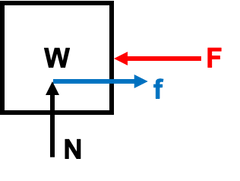If you are moving an object along a surface, friction will need to be considered in your force calculations. The free body diagram above shows an example of a drawer being pushed by a linear actuator. Each drawer slide will have a noticeable amount of friction as they are supporting a perpendicular load (W). As there are two drawer slides, the normal force (N) applied by one of drawer slides will be equal to half of the load (W). Summing the forces and solving for F in this example will result in:

F > u*(0.5*W) + u*(0.5*W) = u*W

Thus, the force you require from the linear actuator needs to be greater than the total force of friction. The tricky part in these cases is determining the coefficient of friction. If you are able to determine the exact coefficient of friction in your application, then you can simply use the above formula to solve for your minimum dynamic force. If you cannot determine coefficient of friction, you can assume it to be equal to 1. This will probably be greater than the actual coefficient of friction, so it is a safe assumption to use to determine the amount of force you require from your linear actuator.

## Two-Dimensional Motion

So far, we have only looked at moving an object along one-axis, but you may require motion in two-axis or at an angle. In these cases, you can still use force summation to determine the dynamic force required but we’ll need to consider multiple axes and make use of some trigonometry. In the example below of pushing an object up a ramp, the direction of motion is at an angle (theta). To simplify our calculations, you can choose to have the one axis be parallel to the direction of motion and the other axis will then be perpendicular, as shown.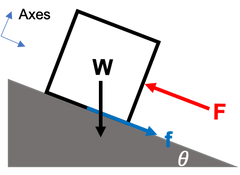Now that the axes are shifted, you will need to divide the weight of the object into two force components by utilizing trigonometry and the slope of the ramp (theta). One of these forces will act against our direction of motion and one will act perpendicular to the surface of the ramp. The normal force, used to determine the friction force, will be equal to the perpendicular component of the weight of the object. Solving the summation of forces to determine F will result in:

F > W*sin(theta) + u*N = W*sin(theta) + u*W*cos(theta)

## Rotational Motion

While linear actuators provide linear motion, they can also be used to provide rotation in applications like opening a lid or hatch. The dynamic force required to provide rotation will need to result in an unbalanced torque rather than an unbalanced force. A torque is a turning force that causes rotation and is equal to the force applied times the perpendicular distance to the point of rotation. So, to cause rotation, a linear actuator must provide a torque greater than the sum of all the torques working against the direction of desired rotation.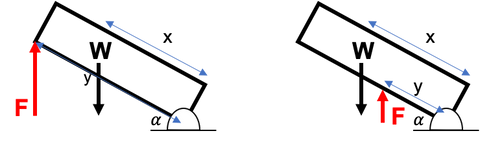The amount of torque your linear actuator applies will depend on two factors, the force applied and the distance from the point of rotation. In the examples above, the summation of torques looks the same:

F*y*cos(alpha) - W*x*cos(alpha) > 0

The distance from the point of rotation to the force from the linear actuator is y, and the distance from the point of rotation to the center of gravity of the hatch is x. As the hatch is at an angle (alpha), we can determine the perpendicular distance to each force by times the distance by the cosine of the angle. Solving for the dynamic force of the linear actuator, F, results in:

F > (W*x)/y

In the case to the left, the dynamic force of the linear actuator, F, can be less or equal to the weight of the hatch, W, because it acts further way from the point of rotation (y > x). While in the case to the right, F will have to be larger than W because F acts closer to the point of rotation, (y < x).In some applications, the force applied by the linear actuator will need to be at an angle like in the image above. This makes the calculations a bit more complicated as the force applied by the linear actuator will need to be broken up in vertical and horizontal components. The free body diagram for the image above is shown below: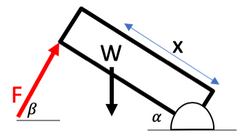The summation of torques for this example is:

((F*cos(beta))*(L*sin(alpha))) + (F*sin(beta))*(L*cos(alpha)) - W*(x*cos(alpha) > 0

Because the force from the linear actuator (F) is applied at an angle (beta), it needs to be broken up into vertical component (F*sin(beta)) and horizontal component (F*cos(beta)), like shown in the ramp example above. The vertical component of the force causes a torque about the hinge as there is a horizontal distance between the force and the hinge; similarly, the horizontal component of the force also causes a torque about the hinge as there is a vertical distance between the force and the hinge. You can determine these distances based on the length of the hatch (L) and the angle of the hatch (alpha), as shown in the previous hatch example. To determine the dynamic force required, you need to solve the above equation for F. Unfortunately, the force from the linear actuator (F) will be a function dependent on the angle of the hatch (alpha). As this angle will change as you open the hatch, the minimum force required from the linear actuator will also change. This means you’ll need to solve the above equation over various angles to find the highest minimum force required to use for your dynamic force specification. This can be even more difficult if the angle at which the force is applied (beta) also changes as the hatch opens, which will mean that it will be a function of the hatch angle (alpha) as well. If you know your mathematics well, you can determine the exact dynamic force requirement you need from your linear actuator. But if not, you can use our handy Linear Actuator Calculator, which is designed just for these difficult situations.

## Static Situations

In a static situation, the summation of forces and the summation of torques will equals zero as there is no unbalanced force or torque causing motion. If you want to ensure your design is stable for a given load or ensure your linear actuator will hold a given load, you can still use the above techniques to ensure all forces and torques are balanced. When checking static situations, you’ll use the static force specification for your linear actuator instead of the dynamic force specification.

Now that you know how to determine how strong your linear actuator needs to be, you can find the right one for your needs in our selection at Firgelli Automations.